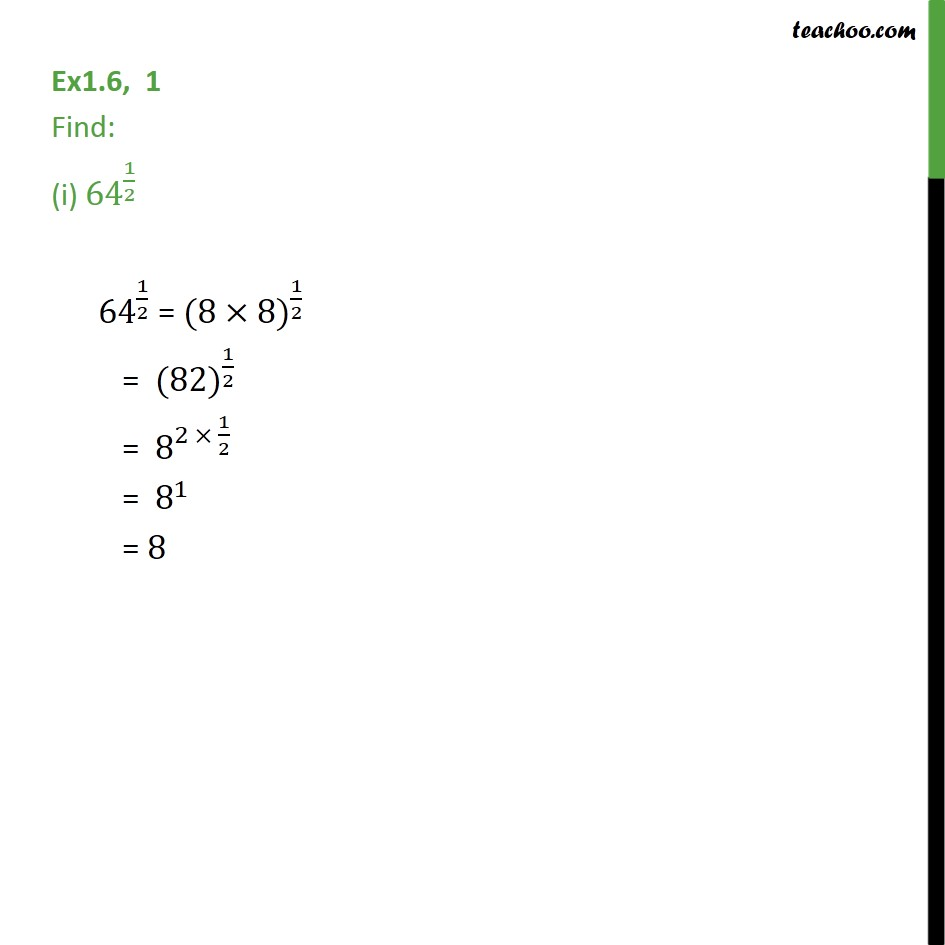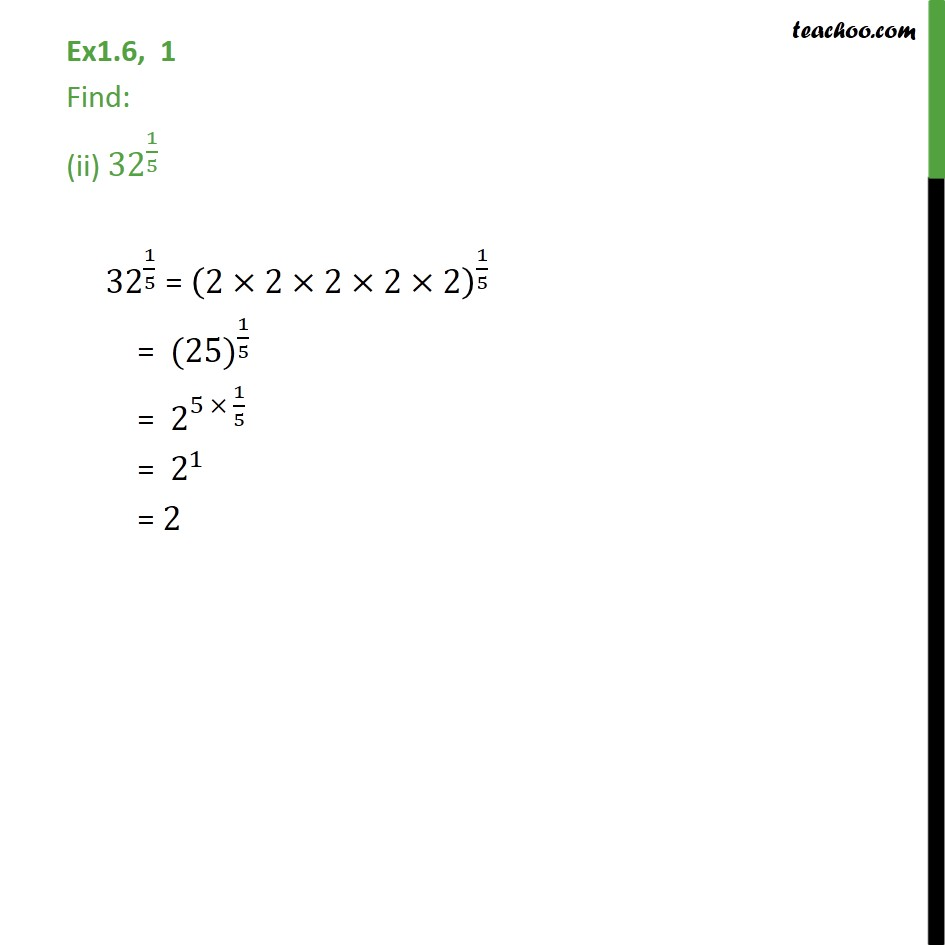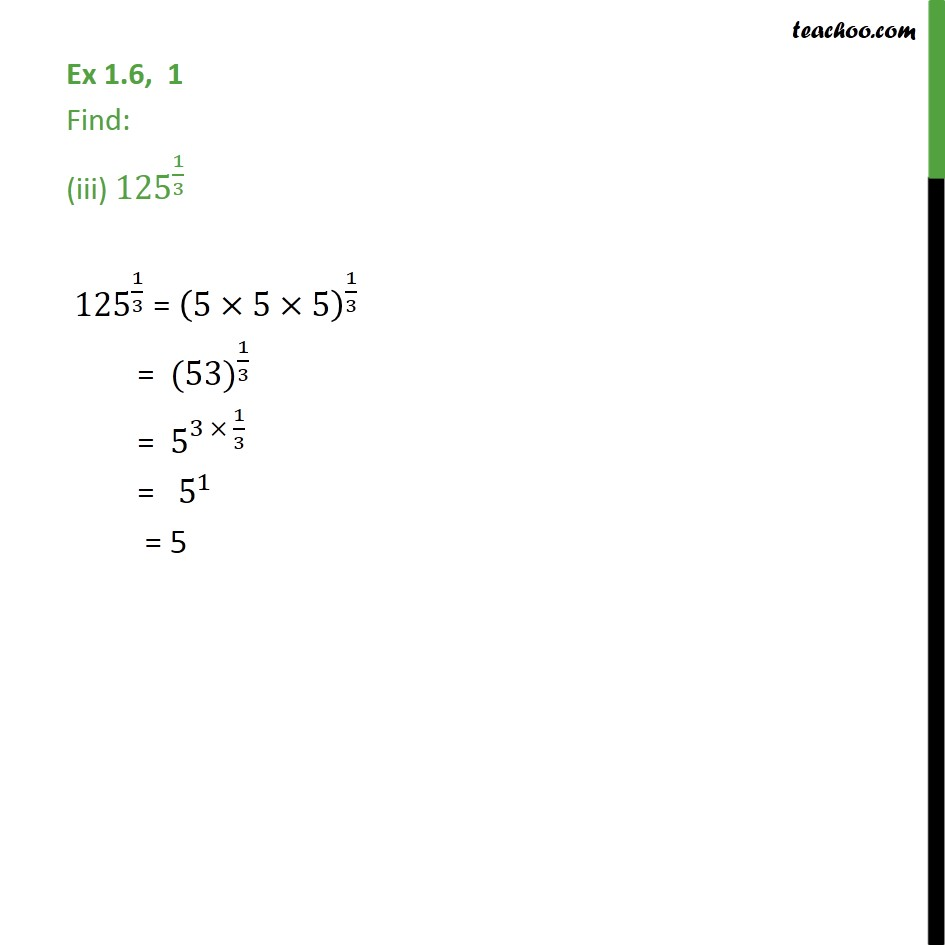Learn All Concepts of Chapter 1 Class 9 - FREE. Check - Chapter 1 Class 9 Maths1. Chapter 1 Class 9 Number Systems
2. Serial order wise
3. Ex 1.6

Transcript

Ex1.6, 1 Find: (i) 〖64〗^(1/2) 〖 64〗^(1/2) = 〖(8×8)〗^(1/2) = 〖 (82)〗^(1/2) = 〖 8〗^(2 × 1/2) = 〖 8〗^1 = 8 Ex1.6, 1 Find: (ii) 〖32〗^(1/5) 〖 32〗^(1/5) = (2×2×2×2×2)^(1/5) = 〖 (25)〗^(1/5) = 〖 2〗^(5 × 1/5) = 〖 2〗^1 = 2 Ex 1.6, 1 Find: (iii) 〖125〗^(1/3) 〖 125〗^(1/3) = (5×5×5)^(1/3) = 〖 (53)〗^(1/3) = 〖 5〗^(3 × 1/3) = " " 〖 5〗^(1 ) = 5

Ex 1.6